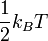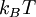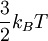Difference between revisions of "Equipartition"This article is a 'stub' page, it has no, or next to no, content. It is here at the moment to help form part of the structure of SklogWiki. If you add sufficient material to this article then please remove the {{Stub-general}} template from this page.
in classical statistical mechanics each degree of freedom that appears quadratically in the energy (Hamiltonian) has an average value of$\frac{1}{2}k_B T$, where$k_B T$ is the thermal energy.
Thus, the thermal energy is shared equally ("equipartitioned") by all these degrees of freedom. This is a consequence of the equipartition theorem, which is very simple mathematically. As an immediate corollary, the translational energy of a molecule must equal$\frac{3}{2}k_B T$, since translations are described by three degrees of freedom.